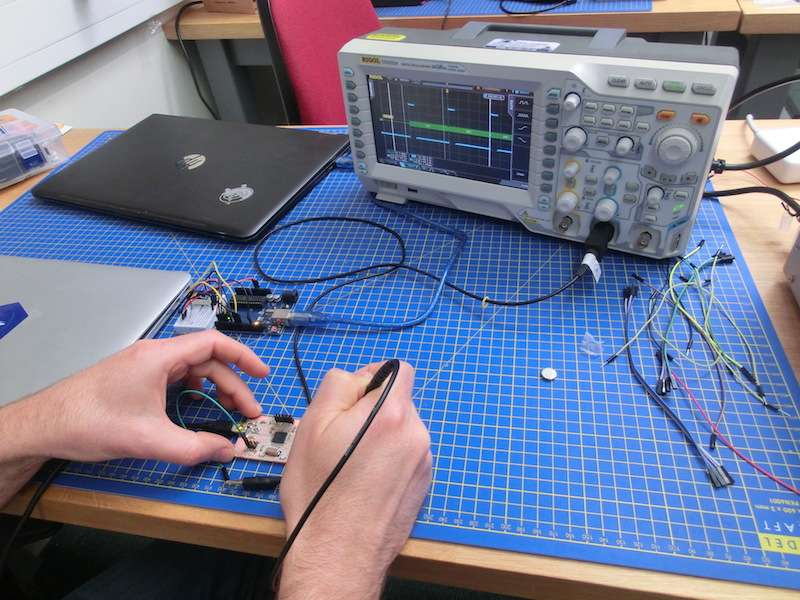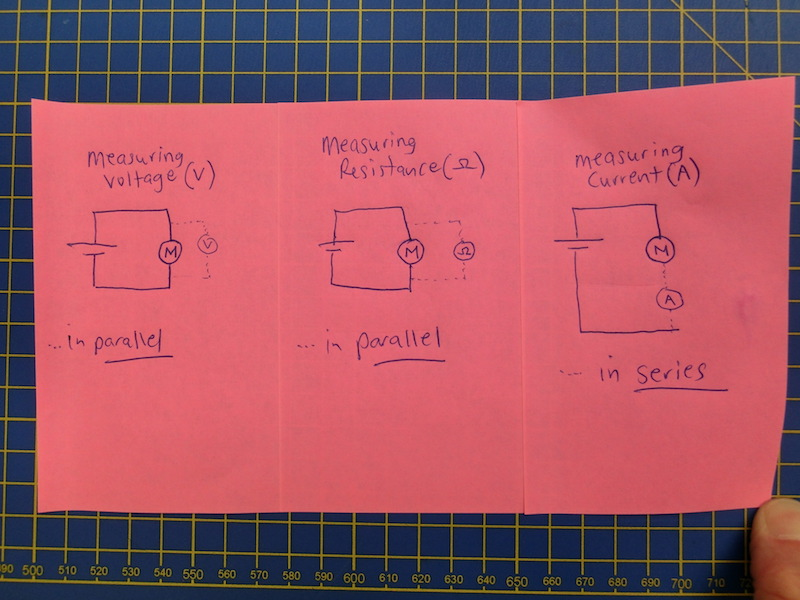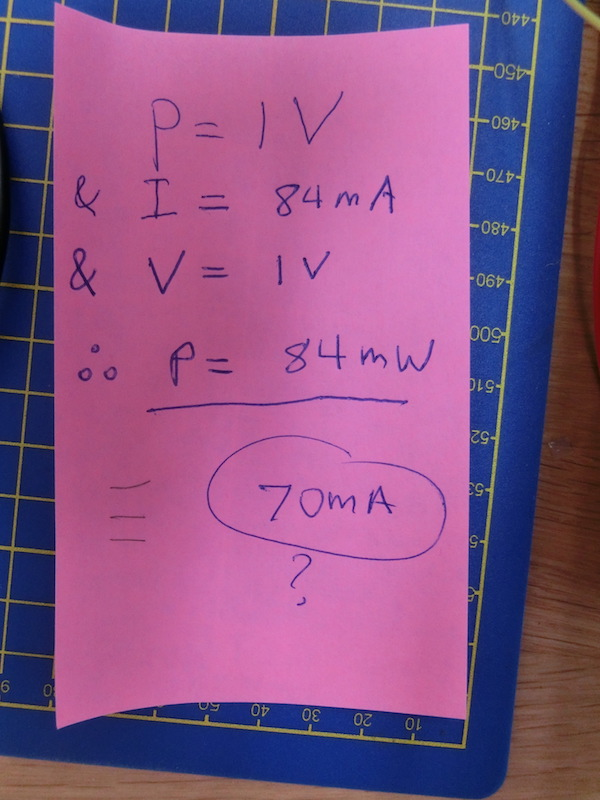# Week 12 - Output devices group assignment

## Measuring the power consumption of an output device

This week we measured the power consumption across a small DC motor. The principle is transferrable to all output devices, measuring voltage, current and resistance is useful and using these you can calculate the power consumed...make sure you get your units right!Power is measured in Watts; Power = Current x Voltage or P = IV

Voltage is measured in Volts...and is measured in parallel to the device/circuit

Resistance is measured in Ohms...and is measured in parallel to the device/circuit

Current is measured in Amps...and is measured in SERIES to the device/circuitHere's a video where we measure the current and voltage and discuss this. It's interesting to note that the voltage provided to the circuit is HIGHER than the voltage lost across the motor. Clearly there is some voltage dropped in the wires...they are resistors after all!

Similarly, the current measured in the power suppy is HIGHER (80mA) when the multimeter is NOT measuring the current than when we are (70mA). Because when we're measuring the current we need to put this in series with the circuit, effectively it is adding in additional resistance to the circuit...again reducing the current slightly